# VBA CreateObjectArticle byExcelMojo Team## What is CreateObject Function in VBA Excel?

VBA CreateObject is a function to reference the ActiveX objects in VBA Excel. CreateObject helps us to reference some of the objects outside of Excel, i.e., MS Word, MS PowerPoint, etc. When we try to reference objects in Excel, we do it in two ways, i.e., Early Binding and Late Binding. For example, look at the following code.

Sub Create_Object()
Dim Power_Point As Object
Set Power_Point = CreateObject(“PowerPoint.Application”)
Power_Point.Visible = True
End Sub

This code will set the object reference for the PowerPoint application outside of Excel.

Early and Late Binding

• Early Binding: If we send the file to someone else containing the MS Word object, they should also set the MS Word object reference in their library before they start using the VBA Code.
• Late Binding: With this method user who receives the file containing the MS Word object need not have to set the object reference in their library. It can be achieved by using the VBA CreateObject function.
###### Key Takeaways
• VBA CreateObject helps us to create references to the required external object through the variable.
• We can reference an object using two methods, and those are Early Binding and Late Binding.
• We can launch Microsoft PowerPoint application through the VBA CreateObject function.
• We can launch the Microsoft Word application through the VBA CreateObject function.
• By using the Shell.Application method, we can launch the Microsoft Edge application.

### Syntax of CreateObject Function in VBA

The following is the syntax of the VBA CreateObject function.

CreateObject (Class As String, [Server Name As String])

• Class: Inthis argument, we need to provide the object that we would like to set the reference using the variable defined earlier.
• [Server Name]: This is an optional argument. If we ignore this argument, it will take local machine reference by default.

### How to Use CreateObject in Excel VBA?

Let us show you a simple example of using the VBA CreateObject function with a step-by-step approach. Assume we must launch the MS Word document from Excel, then we will use the following steps to create an MS Word object from Excel VBA.

1. Open the Visual Basic editor window by pressing the shortcut key “ALT + F11” from your opened Excel worksheet.

2. Start the sub procedure in the Visual Basic Editor code window by naming the macro as follows.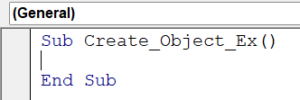3. Define a variable and assign the data type “Object” to it.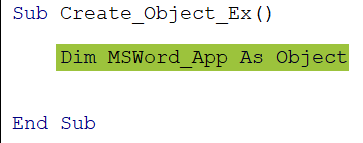Note: Here, we have assigned only the object data type because there is no such data type “MS Word” in Excel. Hence, we must create an object reference with the help of the Object data type and the variable defined.

4. Now, we use the variable “MSWord_App” and assign it the MS Word application object reference using the VBA CreateObject.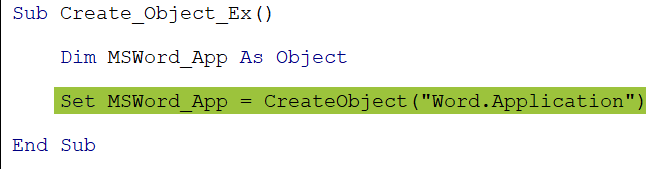Since we are referring to an object variable, we must use the “SET” keyword.

5. The variable “MSWord_App” can now access the MS Word application object. Set the visible property of the MS Word application object to true.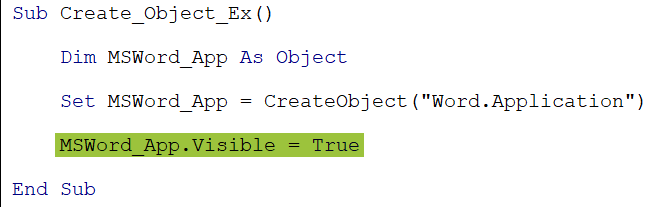The main drawback of this VBA CreateObject is we cannot see the IntelliSense list which will show the properties and methods associated with the MS Word application object.

Let’s run the code and see if the MS Word application opens.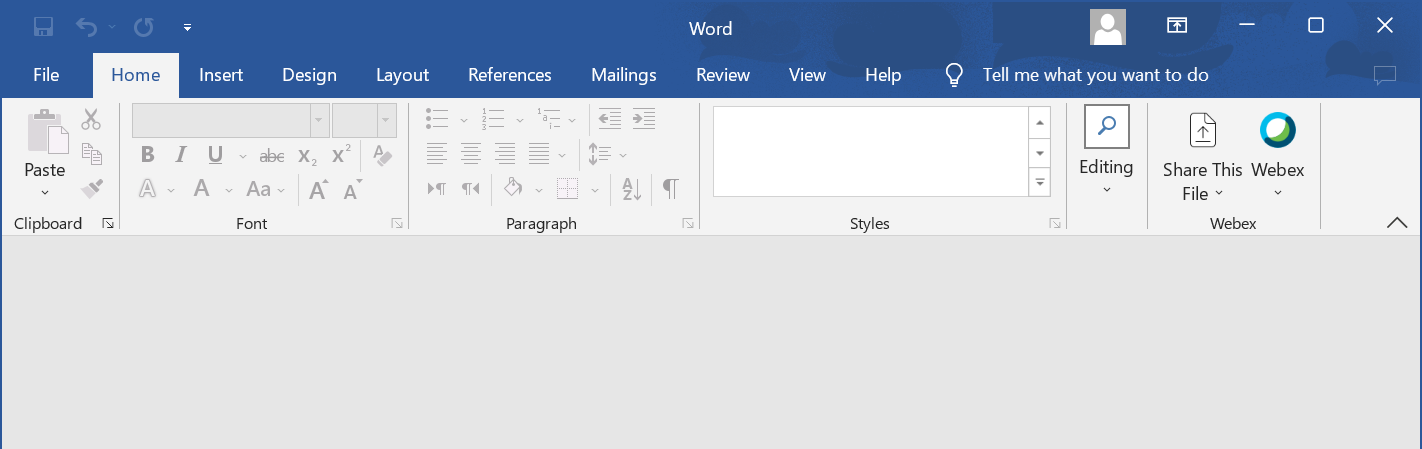### Examples of CreateObject Function in VBA

Let us show you different set of examples of using the CreateObject function in Excel VBA.

#### Example #1 – Invoke MS PowerPoint from Excel Using VBA CreateObject Function

Before we show the importance of using VBA CreateObject, let us look at late binding. As said earlier, in VBA, we can refer to objects in two ways, i.e., Early Binding and Late Binding.

Late binding uses the CreateObject function, while early binding requires setting an object reference in the VBA objects library.

##### Early Binding

Assume we must access the PowerPoint object from VBA. For example, look at the following image.

PowerPoint does not show up in the IntelliSense list when we try to set it to the variable. To make it available in the VBA objects library, we must set the reference to PowerPoint in the Objects library.

• Step 1: Go to “Tools” and click “References.”
• Step 2: It will open the following “References – VBA Project” window.
• Step 3: From this window, choose the “Microsoft PowerPoint 16.0 Object Library.” Note: The version may vary based on the licensing that you have.

Click on Ok. We can now access PowerPoint objects. For example, look at the following image.

The PowerPoint object is visible in the application, and now we can invoke PowerPoint using this reference. For example, look at the following code.

Sub Power_Point_EarlyBinding()
Dim PPT As PowerPoint
Set PPT = New PowerPoint.Application
PPT.Visible = msoCTrue
End Sub

As we can see, we are not using VBA CreateObject here. Instead, we set the reference to the Microsoft PowerPoint library.

One drawback of this method is that if we send the file to someone, they should also set an object reference using the steps above. Otherwise, this code will not work in their system.

One benefit of this method is that the IntelliSense list shows all the properties and methods available under the PowerPoint object.

##### Late Binding

Late binding does not require any object reference setting. The user who receives the file can use the code without modifying anything.

For example, look at the following code.

Sub Power_Point_LateBinding()
Dim PPT As Object
Set PPT = CreateObject(“PowerPoint.Application”)
PPT.Visible = True
End Sub

It will invoke PowerPoint, and we can see the application open in our system.

Once PowerPoint is launched, we can use other properties and methods to build the presentation.

#### Example #2 – Initiate Excel Application from VBA CreateObject

(VBA CreateObject Excel.Application)

Using the VBA CreateObject function, we can initiate the Excel application. Follow the steps listed below.

• Step 1: Define a variable with an object variable assigned to it.
• Step 2: Now, set the Excel application app for the variable defined.
• Step 3: The variable Excel_App variable holds the reference to the Excel application. Using this variable, we can make the Excel application visible.
• Step 4: Next, in the visible Excel application, let’s insert some value in cell A1.

The Excel application has been launched. Let’s run the code. We should see a new Excel workbook and the “Hello Excel VBA” string.

Finally, we must release the variable by setting its value to “Nothing” and quit the Excel application. The following is the completed code to launch the Excel application and finally quit it.

Sub Excel_Application()
‘Declare a variable that holds the object reference
‘Assigning object data type to cause late binding
Dim Excel_App As Object
‘Set the appliction sheet to the variable
Set Excel_App = CreateObject(“Excel.Sheet”)
‘Make the application visible
Excel_App.Application.Visible = True
‘Insert some value to cell A1
Excel_App.Application.Range(“A1”).Value = “Hello Excel VBA”
‘Save the Excel application workbook in the current workbook path folder
‘The name will be Test.xlsx
Excel_App.SaveAs ThisWorkbook.Path & “\Test.xlsx”
‘Quite the Excel application
Excel_App.Application.Quit
‘Set the object variable value to nothing
Set Excel_App = Nothing
End Sub

You can copy this code and use it without any changes.

#### Example #3 – Initiation Microsoft Edge Application Using VBA CreateObject shell.application

We can launch the Microsoft Edge application by using the VBA CreateObject function. Invoking the Edge application requires some shell script to be called inside the macro code.

The following code will launch the Microsoft Edge application object.

Sub Microsoft_Edge()
Dim Msft_Edge As Object
Set Msft_Edge = CreateObject(“Shell.Application”)
End Sub

• Explanation of the Code
• Part 1: This code first sets the Object data type to the variable Msft_Edge.
• Part 2: Then, we will use the VBA CreateObject Edge application option and assign the shell application object to the defined variable.
• Part 3: Finally, we will use the object-referenced variable Msft_Edge, and through this variable, we will execute the ShellExecute and open the Google page using the Microsoft Edge application object. Once we run this code, we will get the following page opened in our Microsoft Edge browser.

Thus, by using the VBA CreateObject shell.application, we can launch the Microsoft Edge application from the CreateObject.

### Important Things to Note

• By default, external application objects are not referenced; Hence, we must manually set the reference for the required object from the toolbar menu.
• The VBA CreateObject function does not show any IntelliSense list. Hence, one must know all the referenced object’s class, properties, and methods.
• If you use the early binding approach (without the VBA CreateObject function), the file receiver also sets the reference for the required application object before they use the code.

1. What is the Difference Between New and CreateObject in Excel VBA?

The new method requires the required object reference to be set from the VBA object library hence causes early binding. The VBA CreateObject function does not require any object reference to be set hence causes late binding.

2. What is the Difference between GetObject and CreateObject in VBA?

CreateObject: This will create an instance of a specified object.
GetObject: This will get an existing instance of an object.

3. Why is VBA CreateObject not Working?

The VBA CreateObject function will not work if the given application name is not accurate or not recognizable by VBA.

This has been a guide to VBA CreateObject. Here we learn to use CreateObject function in Excel VBA, its syntax, early binding & late binding, with examples. You can learn more from the following articles –